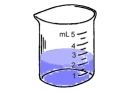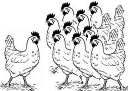Treatment training manual

# Units of measurement

• cubic millimetre – mm3
• millilitre – mL

And two advanced units often used in studies:

• log value – log10
• percentage – %

## Cubic millimetre – mm3A cubic millimetre measures a very small volume. The length, depth and height of the volume are all 1 millimetre (mm) long.

In scientific papers cubic millimetre are sometimes shown as microlitres (µL) or million parts per litre (106/L).

CD4 count is measured as number of cells in a cubic millimetre (mm3). One cubic millimetre is about a drop of blood.

## Millilitre – mLA millilitre measures a volume of liquid.

Viral load is measured as number of copies in a mL.

1,000 mm3 = 1 mL. 1,000 mL = 1 litre.

## Log value – log10

A log value shows a number as a factor of 10.

Log values make it easier to deal with big numbers.

#### Measuring viral load using logs

Viral load is often measured using a log value.

• A 1 log drop in viral load means the amount of HIV has gone down by 90%
• A 2 log drop means it has gone down by 99%

If your viral load was 20,000 then a 1 log drop equals a 10-fold (10 times) decrease to 2,000. A 2 log drop equals a 100-fold decrease (10 x 10 times) to 200.

• A 1 log drop in viral load can mean different things.

A drop from 20,000 to 2,000 and from 2,000 to 200 are both drops of 1 log.

#### Table: log = number

 1 log10 = 10 1.5 log = 30 1.7 log = 50 2 log10 = 100 2.5 log = 300 2.7 log = 500 3 log10 = 1,000 3.5 log = 3,000 3.7 log = 5,000 4 log10 = 10,000 4.5 log = 30,000 4.7 log = 50,000 5 log10 = 100,000 5.5 log = 300,000 5.7 log = 500,000

## Percentage – %A percentage shows a number as a proportion of 100 – the number of parts in 100 parts.

• 1% = 1 in 100

Percentages are often used to compare proportions from different samples.

CD4 cells are sometimes counted as a percentage – the proportion of lymphocytes (white blood cells) that are CD4 cells. A CD4% of 12.5 usually equals a CD4 count of 200 in adults.

Percentages can be made easier to understand by turning them into fractions:

• 20% = 20 in 100 = 20/100

Sometimes percentages are shown as a decimal fraction of 1 (100% = everything you count = 1). To make a percentage into a decimal fraction, divide it by 100.

• 20% = 20/100 = 0.20

#### Table: common percentages and what they mean

• 5% = 0.05 = 1 in 20
• 10% = 0.10 = 1 in 10
• 12.5% = 0.125 = 1 in 8
• 16.5% = 0.165 = 1 in 6
• 20% = 0.20 = 1 in 5
• 25% = 0.25 = 1 in 4 = a quarter
• 33% = 0.33 = 1 in 3 = a third
• 50% = 0.50 = 1 in 2 = half
• 66% = 0.66 = 2 in 3 = two thirds
• 75% = 0.75 = 3 in 4 = three quarters
• 80% = 0.80 = 4 in 5
• 90% = 0.90 = 9 in 10
• 95% = 0.95 = 19 in 20

#### Percentages can deceive!

Percentages show proportions, not actual numbers. It is sometimes difficult to see the whole picture:

• A study reports: 80% of people using our new drug had an undetectable viral load at the end of the study.
• Questions: how many people completed the study? Was it many people, or only a few? Maybe lots of people dropped out because of side effects!?

The report suggests the new medicine worked for 8 in 10 people who took the drug – when it really worked for 8 in 10 people who completed the study.

When looking at percentages, remember to ask ‘percentage of what?’

Last updated: 22 July 2009.